# Section 1 Temperature and Heat Chapter 6 Thermal

• Slides: 30
Download presentationSection 1: Temperature and Heat Chapter 6: Thermal Energy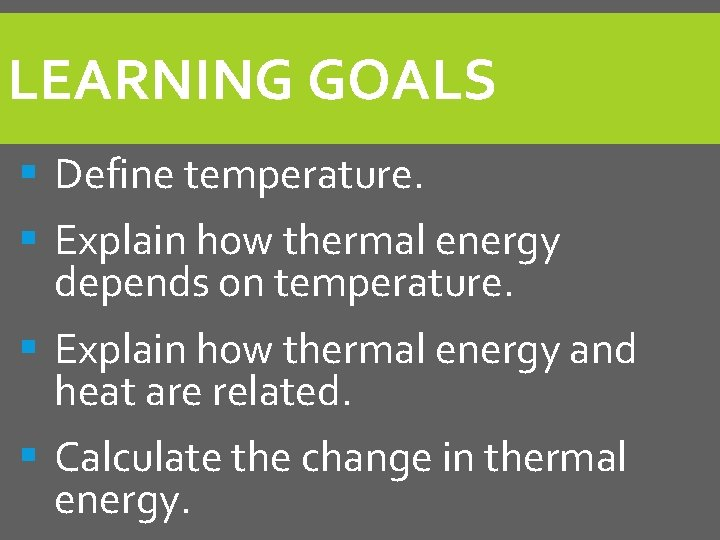LEARNING GOALS § Define temperature. § Explain how thermal energy depends on temperature. § Explain how thermal energy and heat are related. § Calculate the change in thermal energy.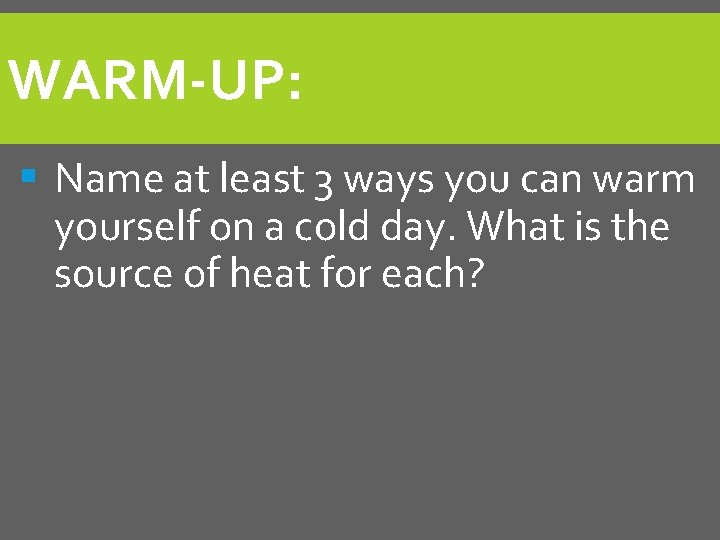WARM-UP: § Name at least 3 ways you can warm yourself on a cold day. What is the source of heat for each?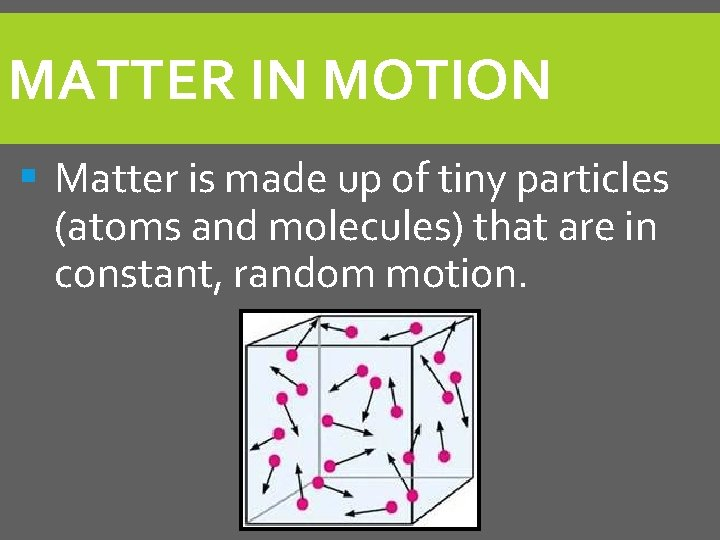MATTER IN MOTION § Matter is made up of tiny particles (atoms and molecules) that are in constant, random motion.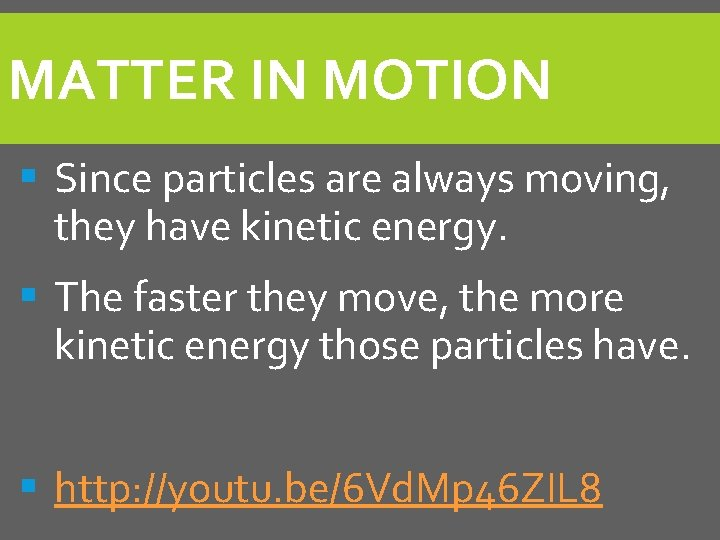MATTER IN MOTION § Since particles are always moving, they have kinetic energy. § The faster they move, the more kinetic energy those particles have. § http: //youtu. be/6 Vd. Mp 46 ZIL 8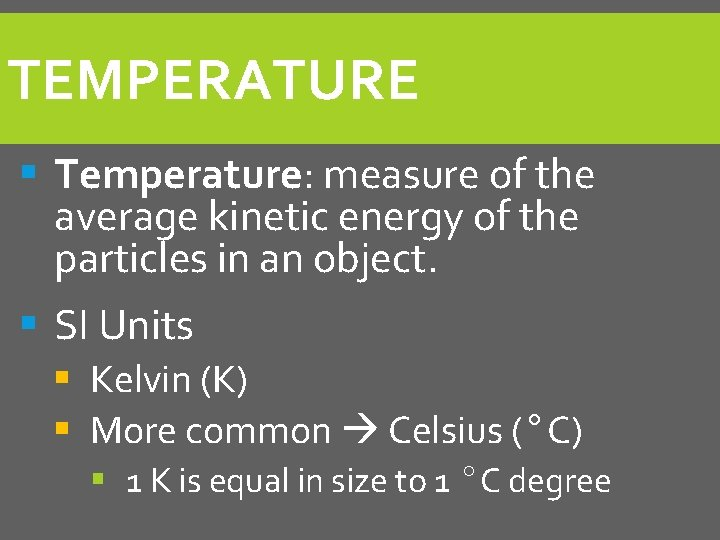TEMPERATURE § Temperature: measure of the average kinetic energy of the particles in an object. § SI Units § Kelvin (K) § More common Celsius (°C) § 1 K is equal in size to 1 °C degree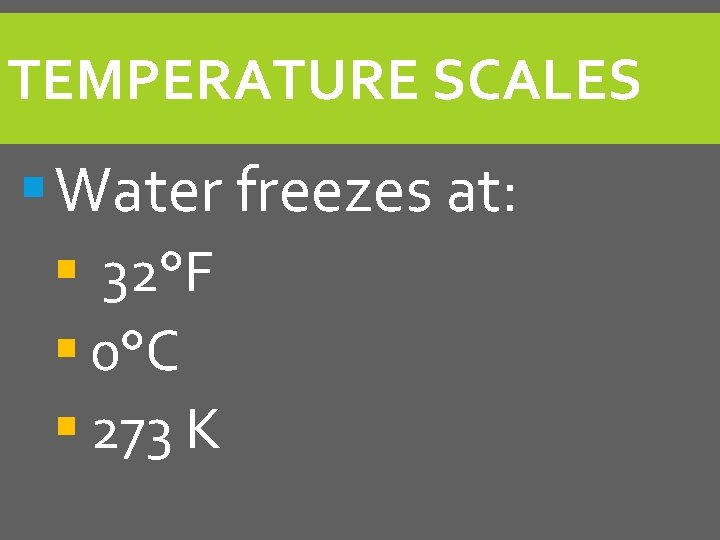TEMPERATURE SCALES § Water freezes at: § 32°F § 0°C § 273 KTEMPERATURE SCALES § Water boils at: § 212°F § 100°C § 373 K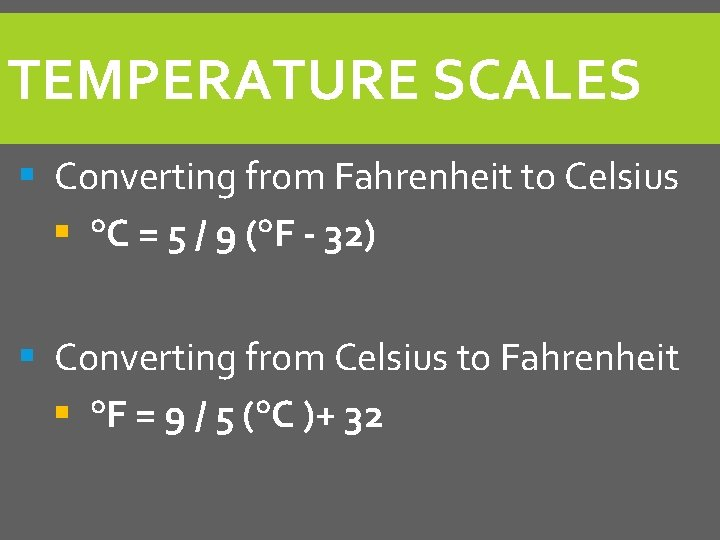TEMPERATURE SCALES § Converting from Fahrenheit to Celsius § °C = 5 / 9 (°F - 32) § Converting from Celsius to Fahrenheit § °F = 9 / 5 (°C )+ 32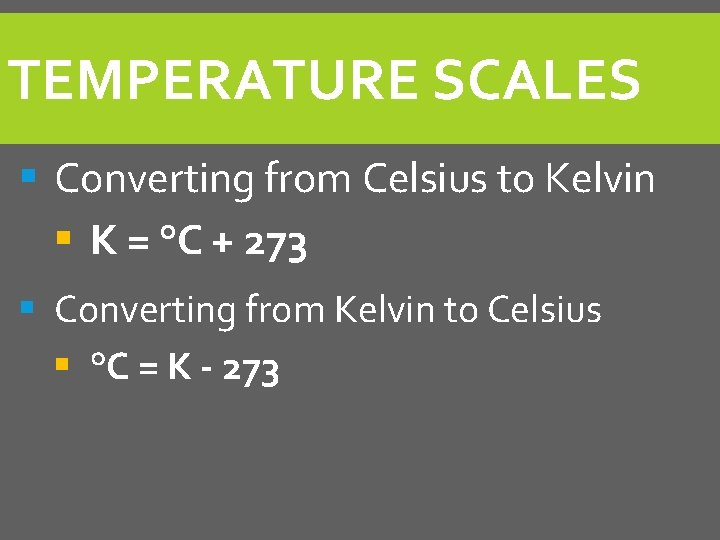TEMPERATURE SCALES § Converting from Celsius to Kelvin § K = °C + 273 § Converting from Kelvin to Celsius § °C = K - 273PRACTICE PROBLEMS § Convert 46 °F to Celsius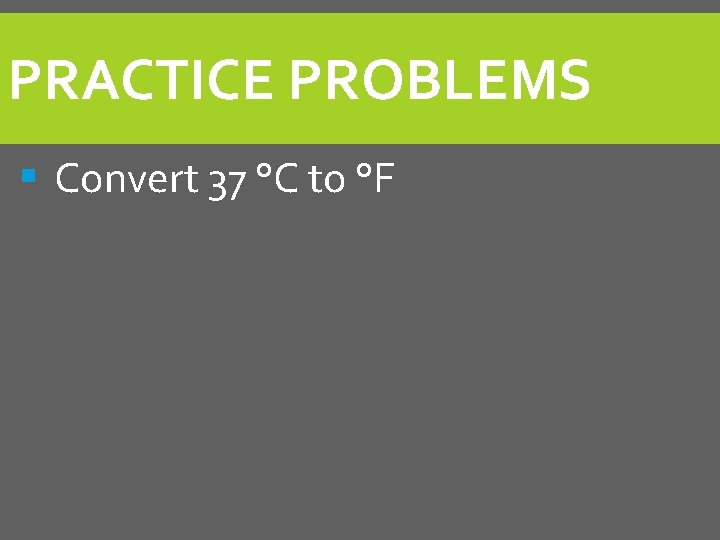PRACTICE PROBLEMS § Convert 37 °C to °FPRACTICE PROBLEMS § Convert 25 °C to Kelvin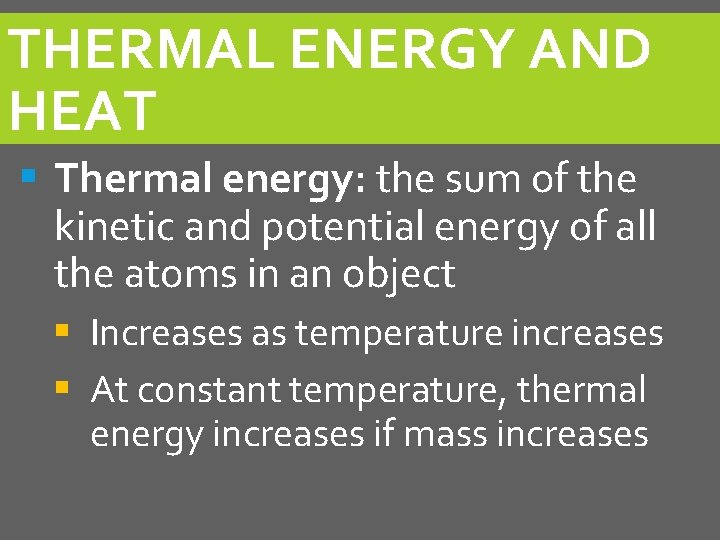THERMAL ENERGY AND HEAT § Thermal energy: the sum of the kinetic and potential energy of all the atoms in an object § Increases as temperature increases § At constant temperature, thermal energy increases if mass increasesTHERMAL ENERGY AND HEAT § Which will cause the greatest increase of temperature of a children’s swimming pool that is at 50°F? § A cup of water at 100°F § A large barrel of water at 80°F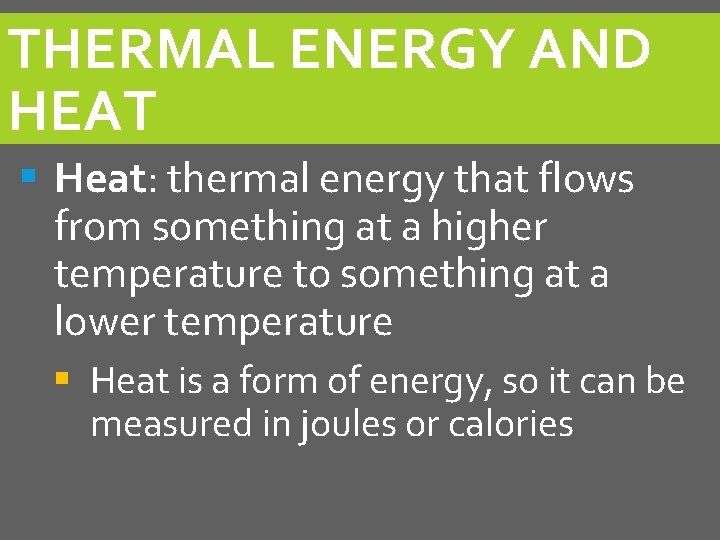THERMAL ENERGY AND HEAT § Heat: thermal energy that flows from something at a higher temperature to something at a lower temperature § Heat is a form of energy, so it can be measured in joules or calories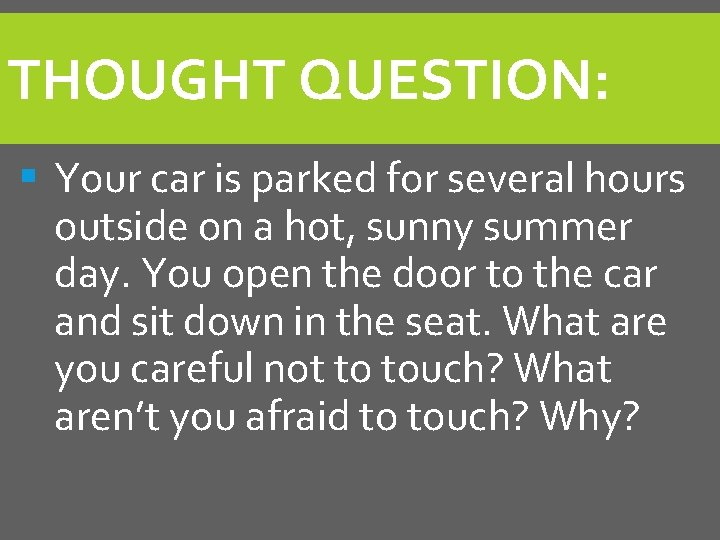THOUGHT QUESTION: § Your car is parked for several hours outside on a hot, sunny summer day. You open the door to the car and sit down in the seat. What are you careful not to touch? What aren’t you afraid to touch? Why?MEASURING HEAT § Heat cannot be measured directly. § However, an increase in temperature indicates that heat is being added. § A decrease in temperature indicates that heat is being removed.MEASURING HEAT § Heat will be measured in calories (cal). § calorie: the amount of heat energy needed to raise the temperature of 1 gram of water by 1°C. § 1 calorie = 4. 184 J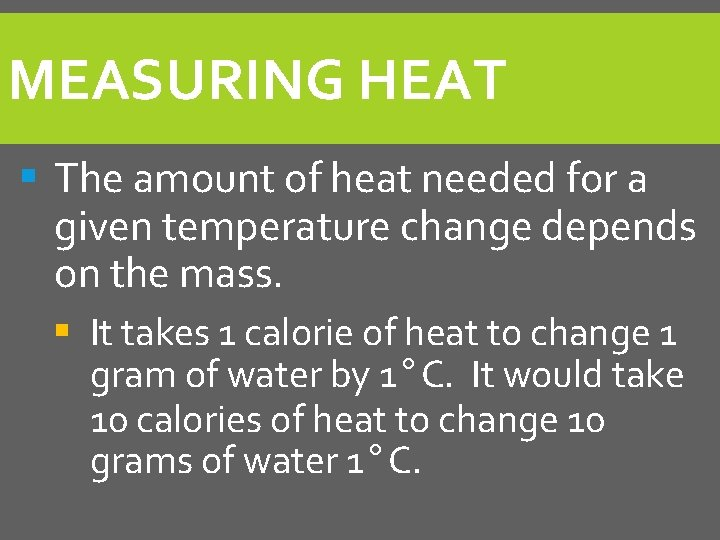MEASURING HEAT § The amount of heat needed for a given temperature change depends on the mass. § It takes 1 calorie of heat to change 1 gram of water by 1°C. It would take 10 calories of heat to change 10 grams of water 1°C.SPECIFIC HEAT § Specific heat: the amount of heat energy that is needed to raise the temperature of 1 gram of a substance by 1°C § Example: The specific heat of water is 1 calorie per gram per degree Celsius. (1. 00 cal/g°C)SPECIFIC HEAT § Heat gained or lost = Mass x Change in Temperature x Specific Heat § q = m x ΔT x C § § q = heat in calories m = mass in g ΔT = change of temperature in °C C = Specific heat in cal/g°C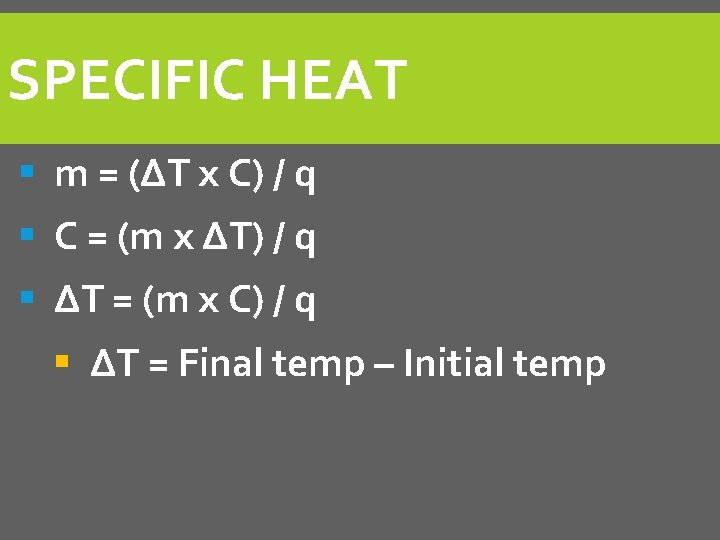SPECIFIC HEAT § m = (ΔT x C) / q § C = (m x ΔT) / q § ΔT = (m x C) / q § ΔT = Final temp – Initial temp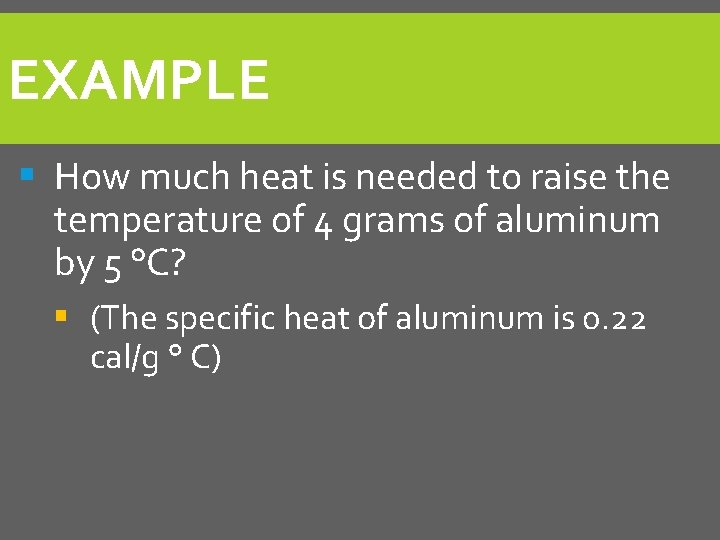EXAMPLE § How much heat is needed to raise the temperature of 4 grams of aluminum by 5 °C? § (The specific heat of aluminum is 0. 22 cal/g ° C)CALORIMETRY § Calorimeter: an instrument used to measure the heat given off by a chemical reaction § Works because within a closed container, the heat lost by one substance must equal the heat gained by another substance.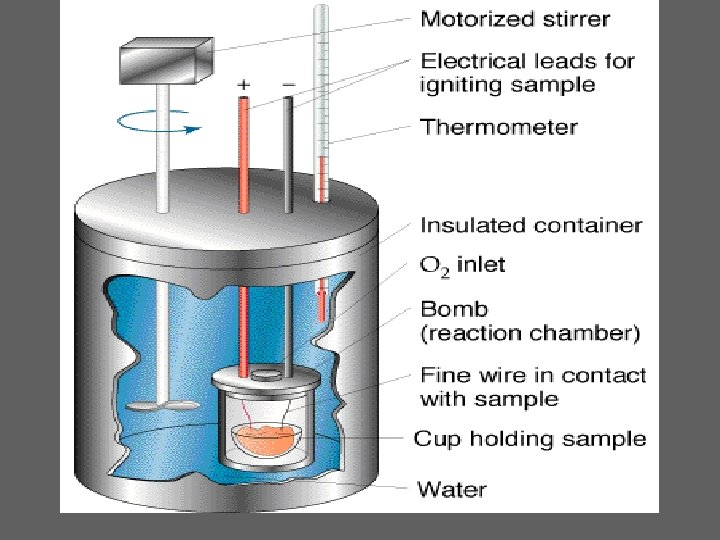CALORIMETRY § Heat energy will not change the temperature of a substance when the heat energy is stored. § Examples: food and gasoline § Heat energy is released when the substances burnCHECK-IN: § Using an arrow, draw the direction of energy transfer between the two objects below: 78°C 89°C